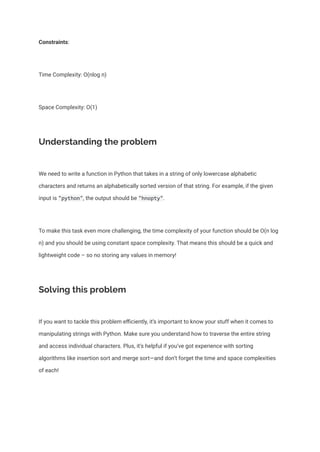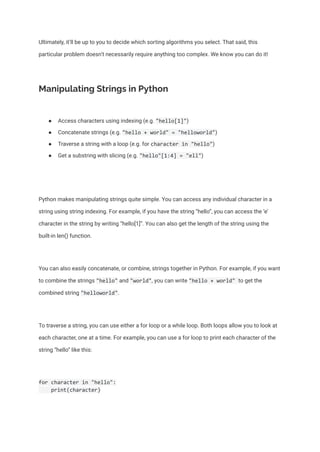Diese Präsentation wurde erfolgreich gemeldet.

# Sort Characters in a Python String Alphabetically

Anzeige
Anzeige
Anzeige
Anzeige
Anzeige
Anzeige
Anzeige
Anzeige
Anzeige
Anzeige
AnzeigeAnzeige
×

1 von 8 Anzeige

# Sort Characters in a Python String Alphabetically

This tutorial will explore how to solve the problem of sorting characters in a Python string in alphabetical order. We'll learn about manipulating strings in Python and discuss how to compare various sorting algorithms. Finally, we'll put the pieces together to create a solution using the merge sort algorithm. Problem-solving can be quite challenging, but with the right approach, we can find an efficient solution with minimal time and space complexity.

#Python #Strings #Sorting #Algorithms #MergeSort #TimeComplexity #SpaceComplexity #Coding #Recursion

This tutorial will explore how to solve the problem of sorting characters in a Python string in alphabetical order. We'll learn about manipulating strings in Python and discuss how to compare various sorting algorithms. Finally, we'll put the pieces together to create a solution using the merge sort algorithm. Problem-solving can be quite challenging, but with the right approach, we can find an efficient solution with minimal time and space complexity.

#Python #Strings #Sorting #Algorithms #MergeSort #TimeComplexity #SpaceComplexity #Coding #Recursion

Anzeige
Anzeige

### Sort Characters in a Python String Alphabetically

1. 1. Python Coding Challenges Python String Challenges Sort Characters in a Python String Alphabetically written by Kal Bartal February 8, 2023 Problem: Given a string, write a function in Python to sort the characters in the string in alphabetical order. Note that the string is composed of only lowercase characters. Example: Input: "python" Output: "hnopty"
2. 2. Constraints: Time Complexity: O(nlog n) Space Complexity: O(1) Understanding the problem We need to write a function in Python that takes in a string of only lowercase alphabetic characters and returns an alphabetically sorted version of that string. For example, if the given input is "python", the output should be "hnopty". To make this task even more challenging, the time complexity of your function should be O(n log n) and you should be using constant space complexity. That means this should be a quick and lightweight code – so no storing any values in memory! Solving this problem If you want to tackle this problem efficiently, it’s important to know your stuff when it comes to manipulating strings with Python. Make sure you understand how to traverse the entire string and access individual characters. Plus, it’s helpful if you’ve got experience with sorting algorithms like insertion sort and merge sort—and don’t forget the time and space complexities of each!
3. 3. Ultimately, it’ll be up to you to decide which sorting algorithms you select. That said, this particular problem doesn’t necessarily require anything too complex. We know you can do it! Manipulating Strings in Python ● Access characters using indexing (e.g. "hello") ● Concatenate strings (e.g. "hello + world" = "helloworld") ● Traverse a string with a loop (e.g. for character in "hello") ● Get a substring with slicing (e.g. "hello"[1:4] = "ell") Python makes manipulating strings quite simple. You can access any individual character in a string using string indexing. For example, if you have the string “hello”, you can access the ‘e’ character in the string by writing “hello”. You can also get the length of the string using the built-in len() function. You can also easily concatenate, or combine, strings together in Python. For example, if you want to combine the strings "hello" and "world", you can write "hello + world" to get the combined string "helloworld". To traverse a string, you can use either a for loop or a while loop. Both loops allow you to look at each character, one at a time. For example, you can use a for loop to print each character of the string “hello” like this: for character in "hello": print(character)
4. 4. You can also use slicing to get a substring from a string. For example, if you wanted to get the substring “ell” from the string “hello”, you could use this code: substring = "hello"[1:4] Comparing Sorting Algorithms ● Insertion sort: Loops through list from left to right and inserts each element into its proper place. Time complexity of O(n^2). ● Merge sort: Divides list into two halves, sorts each half, and then merges them back together. Time complexity of O(n log n). ● Insertion sort more efficient when list is mostly sorted. Sorting algorithms are used to sort a list of items in a certain order. Two of the most common sorting algorithms are insertion sort and merge sort. Insertion sort works by looping through the list from left to right and inserting each element into its proper place until the list has been sorted. This algorithm has a time complexity of O(n^2). Merge sort works by dividing the list into two halves, sorting each half, and then merging them back together. This algorithm has a time complexity of O(n log n). Both insertion sort and merge sort have their pros and cons, so you’ll want to choose the right one for the job. For example, if the list is already mostly sorted, insertion sort may be a better choice.
5. 5. Sorting Characters in a Python String Alphabetically This solution code below is an example of how to solve this problem using the merge sort algorithm. Merge sort is optimal in this case since it has a time complexity of O(n log n) and a constant space complexity. First, we define a recursive function called merge_sort() which will sort the characters in our string. This function takes in a string as an argument and returns the sorted string. from heapq import merge def merge_sort(string): # Base case if len(string) == 1: return string # Split the string in half mid = len(string) // 2 left = string[:mid] right = string[mid:] # Sort the left and right halves left_sorted = merge_sort(left) right_sorted = merge_sort(right) # Merge the sorted halves return merge(left_sorted, right_sorted) Next, we define a function called merge() which merges two sorted halves of our string. This function takes in two strings as arguments and returns a single, sorted string which is composed of both strings. def merge(left, right): merged = ""
6. 6. left_index = 0 right_index = 0 # Compare the left and right strings, character by character while left_index < len(left) and right_index < len(right): if left[left_index] < right[right_index]: merged += left[left_index] left_index += 1 else: merged += right[right_index] right_index += 1 # Add any remaining characters from either string merged += left[left_index:] merged += right[right_index:] return merged Finally, we can solve the problem by calling the merge_sort() function on our string. string = "python" sorted_string = merge_sort(string) print(sorted_string) # prints "hnopty" Time Complexity ● O(n log n) time complexity, same as merge sort algorithm ● Halving process happens log n times ● Overall time complexity O(n log n) The time complexity of this code is O(n log n), which is the time complexity of the merge sort algorithm. This means that our code has the same time complexity as dividing the list into two halves, sorting each half, and merging them back together.
7. 7. The time complexity of O(n log n) is a result of the halving process which occurs whenever we divide our list into two halves. This halving process will occur log n times, which results in an overall time complexity of O(n log n). Space Complexity ● Constant space complexity of O(1) ● No need to store values in memory ● Memory usage is always the same The space complexity of this code is O(1), which is constant space complexity. This means that our code only uses a fixed amount of memory to store intermediate variables, regardless of the size of the input string. This is possible because we have used a recursive approach to solving this problem. In this approach, we don’t need to store any values in memory and so the amount of memory used is always the same. Final Thoughts ● Sharpen coding skills ● Learn recursive solutions ● Analyze time/space complexity
8. 8. Sorting strings can be a challenging but rewarding task. Not only does it help you to sharpen your coding skills, it also teaches you useful techniques, such as recursive solutions and time/space complexity analysis. If you have any questions or need any help understanding this problem, don’t hesitate to reach out and ask.